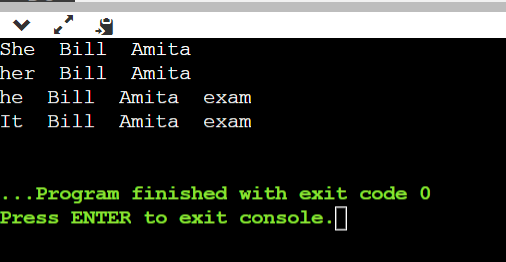# Algorithm to find noun and pronoun words in a paragraph

## Question

It may not be the best way, but we could store each paragraph in a story in a linked list. In fact, we can store an entire story as a linked list of pointers to paragraphs. In this problem, we just need to worry about the paragraph level, though. When you are reading, sometimes you encounter a pronoun (like “her” (object) or “She” (subject)). These words usually refer back to the most recent noun. (NOT ALWAYS!!!). It would be nice to check how far back we have to go for candidate nouns within the last 25 words.

Given an input story like:

Bill and Amita were happy. She got an A on her exam while he got B. It was easier for her.

We want to produce an output that lists each pronoun as we encounter it than a list of the candidate nouns (in order by closeness) like this:
She Amita Bill
her Amita Bill
he exam Amita Bill
it exam Amita Bill

Write an algorithm that accomplishes this task using some kind of linked list. I will grant you a magic function is_noun() that returns true if a word is a noun and false if it is any other word type (It is always correct).

## Summary

In this question, we have been given a paragraph and we have to make two lists of noun words and pronoun words and then we have to append the respective words into those lists. We have to write an algorithm to find a noun and a pronoun word in a paragraph.

## Explanation

We will read the paragraph word by word and check if the current word is noun or pronoun or any other word. If the current word is a noun then we append it to the noun_list and if it is a pronoun then we will append it to the pronoun_list. We have defined the functions { is_noun() and is_pronoun() }  to check if a word is noun or pronoun. After executing the complete program, we will print the output.

## Algorithm

LIST OF CANDIDATE NOUNS(Paragraph):
Allocate memory for noun_list[]
pronoun_list[] for pronoun
Allocate memory for index_noun[]
Allocate memory for index_pronoun[]
n = 0
p = 0
for every word in Paragraph:
if is_noun(word) = true
noun_list[n]=word
index_noun[n]=word number
n=n+1

else if is_pronoun(word) = true
pronoun_list[p]=word
index_pronoun[p]=word number
p=p+1

count=0
Allocate memory for candidate_list[][]
for every x in pronoun_list
append x to candidate_list[count]
for every y in noun_list
if index_pronoun[x]>index_noun[y]
append y to candidate_list[count]
else
break

count=count+1
print(candidate_list)


## Code

#include <bits/stdc++.h>
using namespace std;
/* checking if a word is noun or not */
bool is_noun(string str){
if(str=="Amita" || str=="Bill" || str=="exam")
return true;
else
return false;
}
/* checking if a word is pronoun or not */
bool is_pronoun(string str){
if(str=="She" || str=="her" || str=="he" || str=="It")
return true;
else
return false;
}
/* main function */
int main()
{
/* input paragraph */
string paragraph="Bill and Amita were happy. She got an A on her exam while he got B. It was easier for her.";

string noun_list;
string pronoun_list;
int index_noun;
int index_pronoun;
int n=0, p=0;

istringstream ss(paragraph);
string word;
int x=0;
while(ss>>word){
if(is_noun(word)){
noun_list[n]=word;
index_noun[n]=x;
n++;
}
else if(is_pronoun(word)){
pronoun_list[p]=word;
index_pronoun[p]=x;
p++;
}
x++;
}
for(int i=0;i<p;i++){
cout<<pronoun_list[i]<<"  ";
for(int j=0;j<n;j++){
if(index_pronoun[i]>index_noun[j])
cout<<noun_list[j]<<"  ";
else
break;
}
cout<<endl;
}
}


## Output